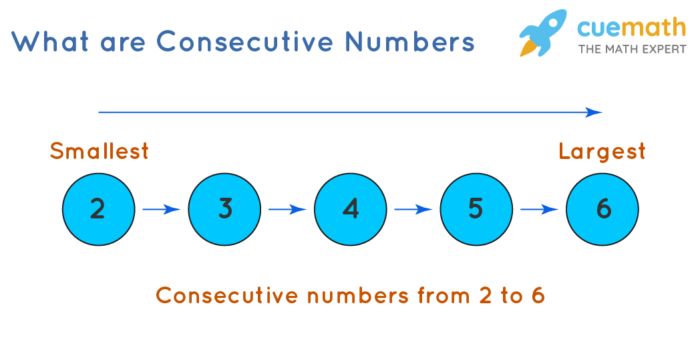# Even and Odd Consecutive Numbers

0
850Patterns and sequences of numbers are essential to understand and perform various tasks. We notice numbers everywhere around us, for calculating objects, representing time, exchanging currency, measuring the temperature, etc. There is virtually nothing that doesn’t include numbers, be it playing games, cooking recipes, counting objects, etc. Consecutive numbers are integers that follow a sequence in which each number is one more than the previous number. These numbers are represented as n, n+1, n+2, and so on, where n is an integer. Without realizing it, we use consecutive numbers in simple counting of objects or for numbering objects.

## Importance of Consecutive Numbers

Learning consecutive numbers enables you to understand how to write an expression or equation to represent a connection between consecutive integers, consecutive even integers, or consecutive odd integers. The concept of consecutive numbers is important to understand in math, primarily in algebra. Solving algebraic problems usually requires knowledge of properties of consecutive odd or even numbers or consecutive numbers that increase by multiples of a certain number.

The concept of consecutive numbers may seem simple, but these consecutive numbers are a little more difficult than they appear. Understanding these consecutive numbers requires the basic skills to identify numbers’ patterns and sequences based on their types and properties. It is a must to identify these consecutive numbers for solving various problems based on them. For instance, when we begin with an even number and each number in the sequence is two more than the previous number, it will be a series of consecutive even integers. In contrast, when we begin with an odd number and each number in the sequence is two more than the previous number, we will get the consecutive odd integers series. Similarly, we can recognize the sequence of various consecutive number series that are multiples of some number and so on.

## Even & Odd Consecutive Integers

The standard consecutive integers are regular consecutive numbers that start from 1 and succeed one by each number. They are represented by n, n+1, n+2, n+3, and so on. These consecutive integers follow the regular counting order. The consecutive integers that follow even and odd counting patterns are known as even and odd consecutive integers.

## Even Consecutive Integers:

These consecutive numbers are the set of integers divisible by two and have a difference of two between them. Even consecutive integers are always divisible by 2. For example, let’s consider ‘n’ is an even number, then the sequence of even consecutive integers will be n+2, n+4, n+6, etc. Even consecutive integers are represented using the equation 2n+2 where n = 0,1,2,3 and so on.

## Odd Consecutive Integers:

These consecutive numbers are a set of integers that consist of odd numbers, and each integer follows the previous integer by a difference of two. For example, if n is an odd number, then the odd consecutive integers are represented as n+2, n+4, n+6, etc., where n is an odd integer. Odd consecutive integers are represented as an expression 2n+1 where n= 0,1,2,…and so on.

Learning consecutive numbers is significant for kids to establish the core foundation of mathematical studies. Although this elementary math topic is considered as easy, learners need to acquire a sound knowledge of this topic through practice. Various learning activities like practice worksheets can help students to gain a deeper understanding of these topics. Cuemath offers educational resources that help students to learn the consecutive numbers and their applications in detail. Such resources are competent in promoting a child’s learnability to strengthen their basic knowledge and problem-solving skills. To find some of these resources, visit www.cuemath.com.